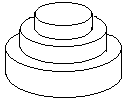## Day 1, Problem 3 - Birthday Cake

July 17th is Mr. W's Birthday, and ACM-THU would like to create for him a birthday cake with volume Nπ, comprised of M cylindrical layers.Counting upwards from the bottom layer, the i-th (1 ≤ iM) layer of cake is a cylinder with a radius of Ri and a height of Hi. When i < M, we require for Ri > Ri+1 and Hi > Hi+1.

To reduce the money spent on icing the cake, we would like to minimize Q, the outer surface area of the cake (not including the bottom surface of the bottommost layer).

Let Q = Sπ. Please write a program that, given N and M, finds a strategy to construct the cake (with appropriate Ri and Hi values) that minimizes the value of S.

Other than Q, all values described above will be positive integers.

### Input Format

The input will consist of two lines. The first line is the integer N (N ≤ 10000), indicating that the volume of the cake is Nπ. The second line of input is the integer M (M ≤ 20), representing the number of levels in the cake.

### Output Format

The output should consist of one line - a positive integer S (if no answer, S = 0).

```100
2
```

### Sample Output

```68
```

Formulas for cylinders:

Volume: V = πR2H
Side surface area: A' = 2πRH
Bottom surface area: A = πR2

Point Value: 20 (partial)
Time Limit: 1.00s
Memory Limit: 64M